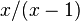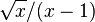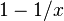# rne

rne(x)
Distribution bounded geometric
Mean approx.$x/(x-1)$
Standard deviation approx.$\sqrt{x}/(x-1)$

rne returns a random number with a bounded geometric distribution. That is, each possible return greater than 1 has a probability that is a fixed fraction of the next lower return, up to a given limit. The fraction is 1 divided by the parameter of rne; thus rne(3) returns 2 one third as often as it returns 1, 3 one third as often as 2, and so on. The maximum return is 5 while the hero's experience level is less than 18; from that point, it is the experience level divided by 3 and rounded down. As experience level cannot exceed 30, the upper bound of rne can never be greater than 10.

A call of rne(3) is used to determine the enchantment of randomly generated weapons, armor, and rings. A call of rne(4) is a component of rnz.

## Mathematical analysis

The effect of experience level on the return from rne is often overstated. Only possible returns greater than 5 are affected, and these are improbable events in any case. Thus, while a level 30 hero could in principle find a random weapon with +10 enchantment, this is a rare event indeed.

Here are the probabilities of each return from rne(3) for experience levels 1 and 30:

Return Level 1 Level 30
1 2/3 2/3
2 2/9 2/9
3 2/27 2/27
4 2/81 2/81
5 1/81 2/243
6 0 2/729
7 0 2/2187
8 0 2/6561
9 0 2/19683
10 0 1/19683

### Description in terms of the Wikipedia article

For the formulae in the Wikipedia article on the geometric distribution, the value of p for a call of rne(x) is the probability that the returned value will be 1; this value is$1-1/x$. The mean and standard deviation for the above infobox are calculated accordingly; but they are approximate, as for the sake of simplicity they give the values for the unbounded geometric distribution, and the return value from rne is bounded.

This page may need to be updated for the current version of NetHack.

It may contain text specific to NetHack 3.4.3. Information on this page may be out of date.

Editors: After reviewing this page and making necessary edits, please change the {{nethack-343}} tag to the current version's tag or {{noversion}} as appropriate.